# Calculator Convert fraction to a decimal

### 3 / 4 = 0.75

Spelled result in words is zero point seven five.

### Calculation steps

1. Divide: 3 / 4 = 0.75
The calculator makes basic and advanced operations with decimals, real numbers and integers. It also shows detailed step-by-step information about calculation procedure. Solve problems with two, three or more decimals in one expression. Add, subtract and multiply decimals step-by-step. This calculator uses addition, subtraction, multiplication or division for calculations on positive or negative decimal numbers, integers, real numbers and whole numbers. This online decimals calculator will help you understand how to add, subtract, multiply or divide decimals.The calculator follows well-known rules for order of operations. The most common mnemonics for remembering this order of operations are:
PEMDAS - Parentheses, Exponents, Multiplication, Division, Addition, Subtraction.
BEDMAS - Brackets, Exponents, Division, Multiplication, Addition, Subtraction
BODMAS - Brackets, Of or Order, Division, Multiplication, Addition, Subtraction.
GEMDAS - Grouping Symbols - brackets (){}, Exponents, Multiplication, Division, Addition, Subtraction.
Be careful, always do multiplication and division before addition and subtraction. Some operators (+ and -) and (* and /) has the same priority and then must evaluate from left to right.

## Decimals in word problems:

• Expanded formWhat is the expanded form of 0.21?
• RatioIncrease in the ratio 20:4 number 18.5.
• Rolls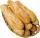Mom bought 13 rolls. Dad ate 3.5 rolls. How many rolls left when Peter yet put two at dinner?
• Ratio v2Decrease in the ratio 12:16 number 13.2.
• Package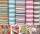The package was 23 meters of textile. The first day sold 12.3 meters. How many meters of textile remained in the package?
• Lowest termsReduce to the lowest terms: 32/124
• Tenths digit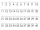For 10.932, which digit is in the tenths place?
• Whole numbersPavol wrote down a number that is both rational and a whole number. What is one possible number she could have written down?
• What is one third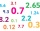What is 1/3 as a decimal? Give your answer rounded to 2 decimal places.
• Sharing chocolateFredericca and her friends share 6 chocolate bars. They *each* get 0.75 of a chocolate bar. How many friends did Fredericca share the chocolate bar with?
• AngieAngie poured 4 cartons of milk, each with 2.4 litres, into a mixing bowl. How many litres of milk were there altogether?
• Double 5Peter was thinking of a number. Peter doubles it and gets an answer of 8.6. What was the original number?
• Equation 11Solve equation: 0=y-1,2.(y-1,5)
• Andy hadAndy had \$5.95 in his pocket. His father gave him another \$3.55. How much does Andy have altogether now?
• Bureaucracy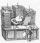23 people attended the office. 9 of them completed the questionnaire. What is the percentage of all people?
• Zhiwei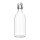Zhiwei wants to divide 19 liters of water equally into 6 pots. Find the volume of water in each pot?
• Rounding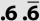Double round number 727, first to tens, then to hundreds. (double rounding)